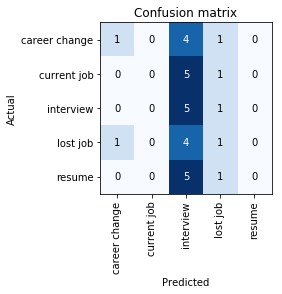# Same code (no randomization/shuffling) produces different results?

Hello. I’m trying to create a classifier that categorizes different Yelp reviews about career coaching businesses. I have around 110 labeled reviews.

The first time I ran the code, I got a max accuracy of 20%. The next time I ran it, I got a max accuracy of 66%. This is a very extreme example, but I’m commonly getting disparate accuracies by running the exact same code (that, as far as I can tell, involves no randomization). Here’s the code:

``````data_clas = (TextList.from_df(df=clas_df, path=path, vocab=data_lm.vocab)
.split_by_idx((range(0,30)))
.label_from_df(cols=1)
.databunch(bs=8))

learn = text_classifier_learner(data_clas, AWD_LSTM, drop_mult=0.5, metrics=[accuracy, AUROC()])

learn.fit_one_cycle(1, 2e-2, moms=(0.8,0.7))

learn.freeze_to(-2)
learn.fit_one_cycle(1, slice(1e-2/(2.6**4),1e-2), moms=(0.8,0.7))

learn.freeze_to(-3)
learn.fit_one_cycle(1, slice(5e-3/(2.6**4),5e-3), moms=(0.8,0.7))

learn.unfreeze()
learn.fit_one_cycle(10, slice(1e-3/(2.6**4),1e-3), moms=(0.8,0.7),
callbacks=[SaveModelCallback(learn,monitor='accuracy',mode='max')])
``````

The first time through, I ran all of the code shown above, and got the following confusion matrix (20% max accuracy):The second time through, I didn’t run the first four lines (I just started by recreating the learner and then ran through all the training code). I got the following confusion matrix (66% max accuracy):I assume in the first case the model is getting itself stuck in some sort of local minima; why does this sometimes happen? I see a lot of the time that the first few cycles have the model predicting all in one or two classes.

Upon running the code 3 more times, I ended up with following max accuracies: 56%, 70%, 60%. It seems to be trending around this general area, but a 14% difference is still quite large. I’ve seen similar cases around other accuracy levels as well.

Is the reason for this variation just that I have a small amount of data (so the initial starting weights have a bigger impact/variation), or is it something else?

Additionally, is it valid to go through this same code many times to save the highest accuracy model? I’m trying to use this model to predict the distribution of unlabeled reviews. Another concern is that certain errors are more preferable to others; for example, I would rather have lost job be misclassified as career change than as current job, since when you lose your job it’s just you being forced into a career change.

Suppose one of the times that I train the model, it ends up commonly misclassifying lost job as career change (like in the second confusion matrix picture). Another time I train the model, it commonly misclassifies lost job as current job (what happened in the 70% accurate model).

Am I allowed to choose the model that I like best and assume that its tendencies will translate when predicting unlabeled reviews? Or is this invalid since the differences were just due to nuances in the training process?

Also, if anyone has any semi-supervised learning resources/guides they’ve used, that would be very useful.

Hi,

When you created the ‘learn’ with this line ‘text_classifier_learner(…)’, your neural network is initialized with random weights.

Then with your 1. training call (1. of this line ‘learn.fit_one_cycle(…)’) the network’s weights are updated to be better to the task - that’s the training/learning.

As I saw you gradually unfreeze the network’s layers then train a bit more the network
(the pairs do this: freeze_to & fit_one_cycle - actually here the freeze_to() do the unfreezing, and because we use -2, then -3, etc more and more layers become free for training - while they were frozen, their weights cannot be updated/changed).

So when you don’t run the 1. 4 lines of code, you do not create a new learner, but the learner is still in the memory with the learnt weights up to this point,
and then when you call the other lines you train the already existing learner even further, that’s why it got better and better accuracy - and that’s what you wantYour confusion matrix also shows that when you got 66% accuracy with more training instead of 20% of the beginning of the training, the network actually learned something, because the matrix’s diagonal finally got good numbers (so the network predicted well the actual targets)

If you want to see the learning process more clearly you can use 1 more parameter on the learn.fit_one_cycle(…), the cbs parameter.
Like this:
cbs = [ShowGraphCallback()]
learn.fit_one_cycle(1, slice(5e-3/(2.6**4),5e-3), moms=(0.8,0.7), cbs=cbs)

Now you can see where your network is in the process, what steps it does, so you also see it is at the beginning or after some training yet.

Yes, it is valid to save your best model after you tried to train it many times, so you can save it with learn.export() or as you did it with SaveModelCallback - it’s a good idea to check if your network is overfitting or not and how many times you try to pick the ‘best’ one, because it also can be a biased pick if you try so many times.

When certain errors are more preferable to others then you can use a weighted loss and specifically set the loss weights for the targets accordingly instead of use the standard loss.
For example I used the CrossEntropyLoss for image classification and when I needed the weighted version, just gave it the loss weight tensor as its 1. parameter (https://pytorch.org/docs/master/generated/torch.nn.CrossEntropyLoss.html)
and I set the weights differently for the classes.
You use text classification so you need to weight your text specific loss, but the idea is the same ^^

I hope I explained the mystery and it is not a mystery anymore